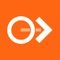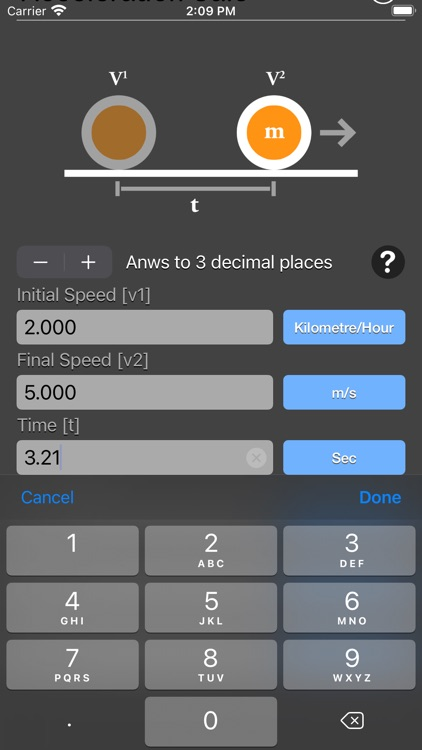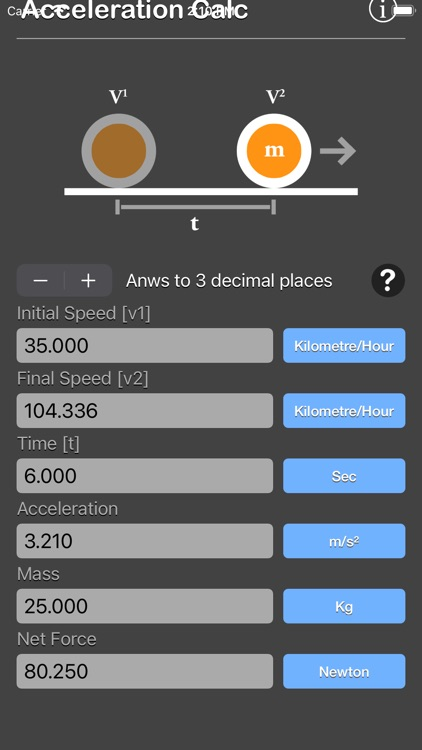## Acceleration Calculator are physic/math calculator to find initial speed, final speed, time, acceleration, mass or force fast and easy# Acceleration Calculator Plus

by NitrioAcceleration Calculator are physic/math calculator to find initial speed, final speed, time, acceleration, mass or force fast and easy.### App Details

Version
1.1
Rating
NA
Size
8Mb
Genre
Utilities Productivity
Last updated
December 22, 2020
Release date
June 3, 2018

### App Screenshots### App Store Description

Acceleration Calculator are physic/math calculator to find initial speed, final speed, time, acceleration, mass or force fast and easy.

Acceleration, in physics, is the rate of change of velocity of an object.

Features:
- Instant calculation
- Result are copy able to other app
- Formula are include as reference
- Support up to 16 decimal place
- Support various unit for each input

The acceleration of a moving object can be determined by using the following formula:
a = (v2-v1) / t
Where:
t: time, in Second
v1: the initial velocity of the object, in m/s
v2: the final velocity of the object, in m/s
a: the acceleration of the object, in m/s2

Newton's Second Law of Motion states that the acceleration of an object as produced by a net force is directly proportional to the magnitude of the net force, in the same direction as the net force, and inversely proportional to the mass of the object.

Newton's Second Law formula:
F = m * a
Where:
F: the magnitude of the net force, in N
m: the mass of the object, in Kilogram
a: the acceleration of the object, in m/s2

In physics, acceleration is the rate of change of velocity of an object with respect to time. An object's acceleration is the net result of any and all forces acting on the object, as described by Newton's Second Law. The SI unit for acceleration is metre per second squared (m s−2). Accelerations are vector quantities (they have magnitude and direction) and add according to the parallelogram law. As a vector, the calculated net force is equal to the product of the object's mass (a scalar quantity) and its acceleration.

For example, when a car starts from a standstill (zero relative velocity) and travels in a straight line at increasing speeds, it is accelerating in the direction of travel. If the car turns, an acceleration occurs toward the new direction. In this example, we can call the forward acceleration of the car a "linear acceleration", which passengers in the car might experience as a force pushing them back into their seats. When changing direction, we might call this "non-linear acceleration", which passengers might experience as a sideways force. If the speed of the car decreases, this is an acceleration in the opposite direction from the direction of the vehicle, sometimes called deceleration. Passengers may experience deceleration as a force lifting them forwards. Mathematically, there is no separate formula for deceleration: both are changes in velocity. Each of these accelerations (linear, non-linear, deceleration) might be felt by passengers until their velocity (speed and direction) matches that of the car.

*This is a universal app that work for both iPhone and iPad.

Thanks for your support and do visit nitrio.com for more apps for your iOS devices.

Disclaimer:
AppAdvice does not own this application and only provides images and links contained in the iTunes Search API, to help our users find the best apps to download. If you are the developer of this app and would like your information removed, please send a request to takedown@appadvice.com and your information will be removed.